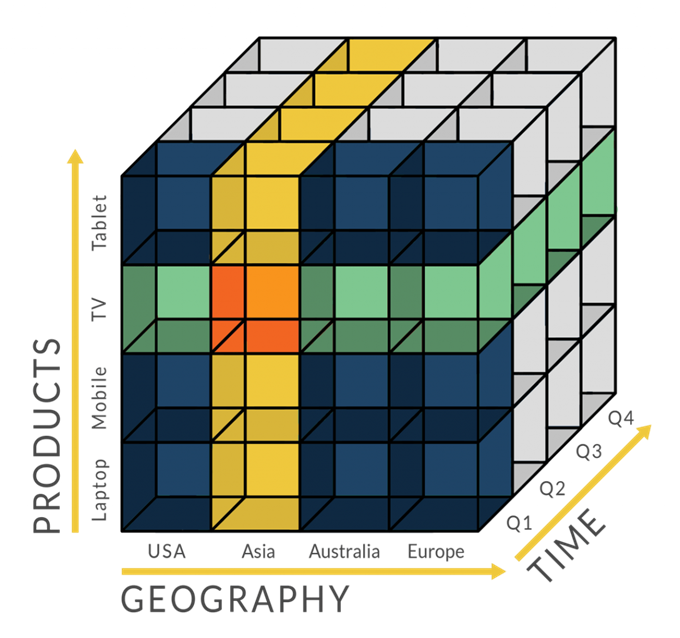# 使用Excel搭建推荐系统

## 学习内容

• 构建帮助赢得 100 万美元 Netflix 奖金的算法版本 SVD++ 背后的确切步骤。
• 机器如何实际学习（梯度下降）。即使您从未告诉过 Netflix，也可以观看 Netflix 了解您的电影品味。
• 超参数调优。了解如何调整模型输入 （学习率、L2 正则化、# of epochs、权重初始化）以获得更好的预测。
• 模型评估和可视化。了解训练数据和测试数据之间的区别，如何防止过度拟合，并了解如何可视化模型的特征。

• 第一部分：模型概览
• 第二部分：观看魔法秀（权重初始化和训练）
• 第三部分：魔法揭秘（梯度下降、导数）。我将逐步讲解机器学习魔法背后的数学，我将使用实数作为例子代入批量梯度下降的公式（不会使用“宏”或者Excel求解器之类的东西隐藏细节）。
• 第四部分：模型评估和可视化

## 推荐系统简介

### 协同过滤

• 隐式（Binging）—— 整个周末，Ross和Rachel都沉溺于老剧《老友记》。尽管他们没人点赞，但我们相当确定他们喜欢《老友记》（以及他们可能有点自恋）。
• 显式（喜欢）—— Ross和Rachel都点了赞。

### 100 万美元的赢家

2009年，Netflix奖励了一队研究人员一百万美元，这个团队开发了一个算法，将Netflix的预测精确度提升了10%. 尽管获胜算法实际上是超过100种算法的集成，SVD++（一种协同过滤算法）是其中最关键的算法之一，贡献了大多数收益，目前仍在生产环境中使用。

### SVD++ 关键思想

• “奇异值”（电影评分）可以“分解”或由一组隐藏的潜在因素（用户偏好和电影特征）决定，这些潜在因素直观地代表了类型、演员等。
• 潜在因素可以使用梯度下降和已知的电影评分迭代学习。
• 用户/电影偏见会影响某人的评分，并且也会被学习。

## 第一部分：模型概览

### 评价预测公式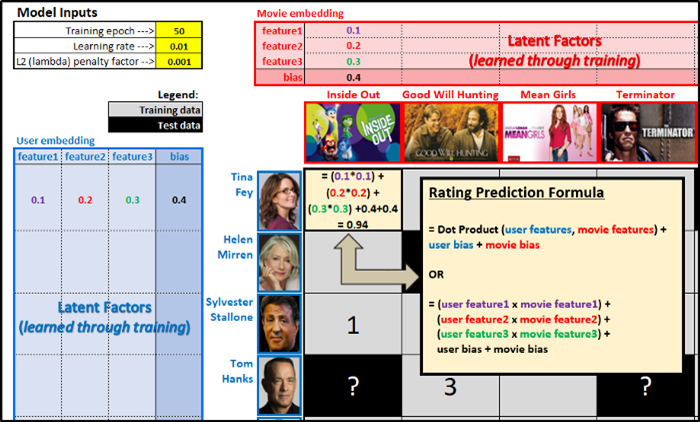$$\hat{r}_{i,j}=((u_1 m_1)+(u_2 m_2)+(u_3 m_3)+u_{bias}+m_{bias})$$

• $\hat{r}_{i,j}$表示用户i对电影j的预测评价
• $u_1$、$u_2$、$u_3$为用户潜因子
• $m_1$、$m_2$、$m_3$为电影潜因子
• $u_{bias}$为用户偏置
• $m_{bias}$为电影偏置

• 直觉上说，这些特征表示类型、演员、片长、导演、年代等因素。尽管我们并不清楚每项特征代表什么，但是当我们将其可视化后（见第四部分）我们可以凭直觉猜测它们可能代表什么。
• 出于简单性，我使用了3项特征，但实际的模型可能有50、100乃至更多特征。特征过多时，模型将“过拟合/记忆”你的训练数据，难以很好地推广到测试数据的预测上。
• 如果用户的第1项特征（让我们假定它表示“喜剧”）值较高，同时电影的“喜剧”特征的值也很高，那么电影的评价会比较高。

### RMSE —— 评估预测精确度

RMSE = Root Mean Squared Error （均方根误差）

RMSE是一个数字，尝试回答以下问题“平均而言，预测评价和实际平均差了几颗星（1-5）？”

RMSE越低，意味着预测越准……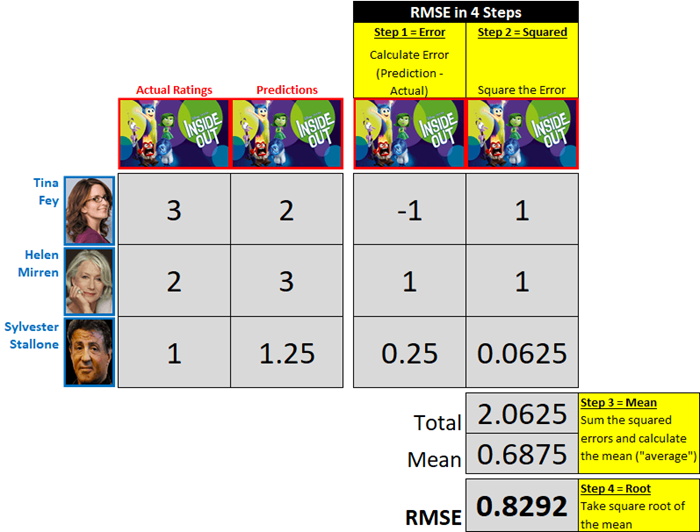• 我们只在意绝对值差异。相比实际评分高估了1分的预测，和相比实际评分低估了1分的预测，误差相等，均为1。
• RMSE是误差同数量级的平均，而不是误差绝对值的平均。在我们上面的例子中，误差绝对值的平均是75（1 + 1 + 0.25 = 2.25，2.25 / 3 = 0.75），但RMSE是0.8292. RMSE给较大的误差更高的权重，这很有用，因为我们更不希望有较大的误差。

### 超参数调整

• 训练epoch数—— 1个epoch意味着整个训练集都过了一遍
• 学习率—— 控制调整权重/偏置的速度
• L2（lambda）惩罚因子—— 帮助模型预防过拟合训练数据，以更好地概括未见测试数据。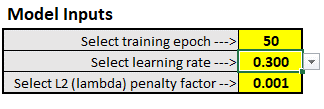## 第二部分：观看魔法秀（权重初始化和训练）

• 他到底是怎么知道我会在52张牌中选这张的呢？
• 等等，他刚刚是不是浮空了？

### 抽一张卡，随便抽一张（权重初始化）

• 简单—— 用户特征我随机选择了1、0.2、0.3，剩下的特征都分配0.1.
• Kaiming He—— 更正式、更好的初始化方法，从高斯分布（“钟形曲线”）中随机抽样作为权重，高斯分布的均值为零，标准差由特征个数决定（细节见后）。

### 观赏魔术（查看训练误差）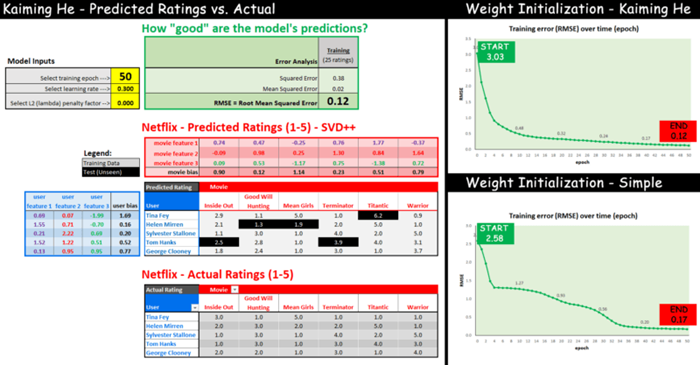### Kaiming He权重初始化

$$W_l \sim \mathcal{N}(0, \sqrt{\frac{2}{n_l}}) \text{and} \mathbf{b}=0$$

## 第三部分：魔法揭秘

• 第 1 步 – 定义成本/损失函数以最小化和初始化权重
• 第 2 步 — 计算预测
• 第 3 步 – 计算梯度（相对于每个权重的成本变化）
• 第 4 步——在最小化成本的方向上更新每个权重“一点点地”（学习率）
• 第 5 步 — 重复第 2-4 步

### 定义最小化的代价函数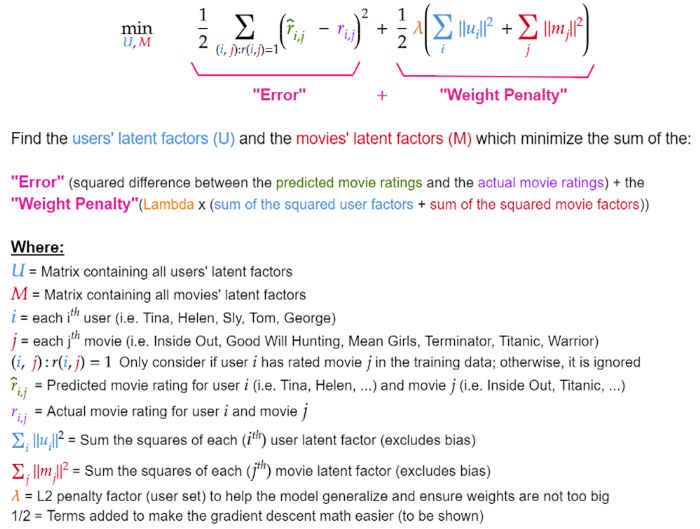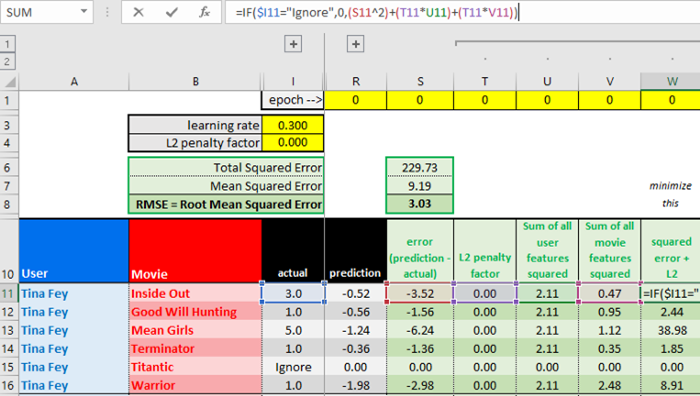L2正则化和过拟合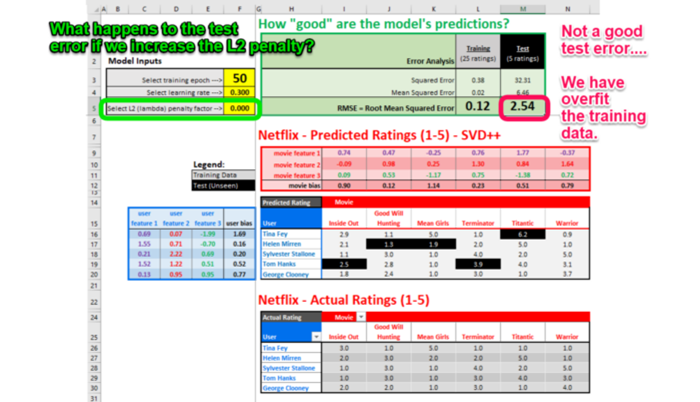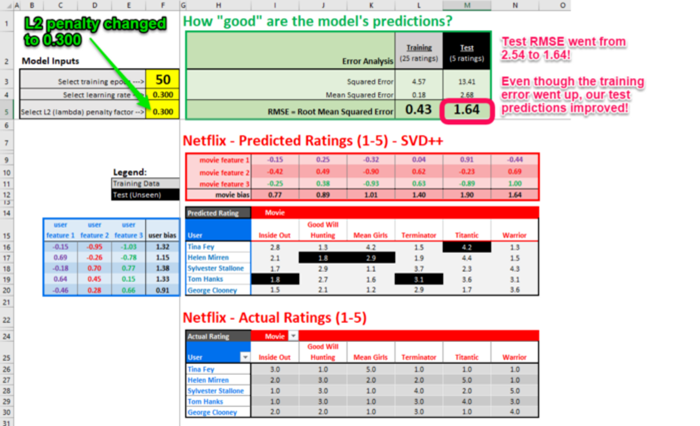### 计算预测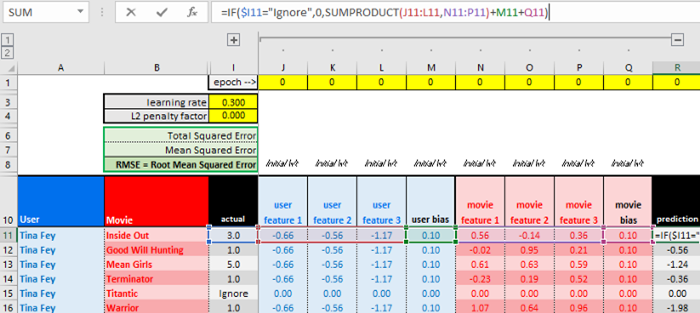### 计算梯度

“移动一点点”具体移动多少，取决于学习率。在得到梯度（3.3）之后，会用到学习率。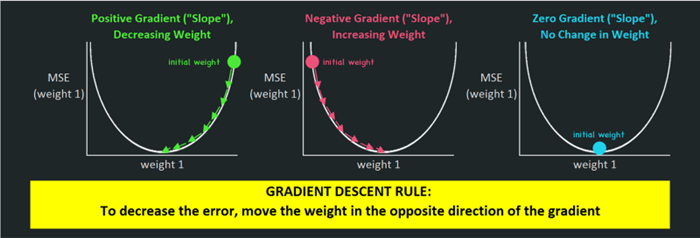1.1整理代价目标函数，取代价在Tina Fey的第一个潜因子（$u_1$）上的偏导数。

$$\frac{\partial J(cost)}{\partial u_1}=\frac{1}{2} \sum_{(i, j): r(i,)=1}(\hat{r}_{i, j}-r_{i, j})^2+\frac{1}{2} \lambda(\sum_i\left\|u_i\right\|^2+\sum_j\left\|m_j\right\|^2)$$

1.2整理预测评价函数，改写为用户潜因子的平方和加上电影潜因子的平方和

$$\frac{\partial J}{\partial u_1}=\frac{1}{2} \sum(((u_1 m_1)+(u_2 m_2)+(u_3 m_3)+u_{bias }+m_{bias})-r_{i, j})^2+ \frac{1}{2} \lambda \sum(u_1^2+u_2^2+u_3^2)+\frac{1}{2} \lambda \sum (m_1^2+m_2^2+m_3^2)$$

1.3将公式每部分中的$u_1$视为常数，取$u_1$在公式每部分的代价上的偏导数。

1.3.1（Part 1 of 3）应用“链式法则”以得到偏导数。链式法则意味着我们将((外层函数的导数)*内层函数)* (内部函数的导数)

$$\begin{gathered}=\frac{1}{2} \sum(((u_1 m_1)+(u_2 m_2)+(u_3 m_3)+u_{bias}+m_{bias})-r_{i, j})^2 \leftarrow \text { power rule} \\=2 \times \frac{1}{2}(((u_1 m_1)+(u_2 m_2)+(u_3 m_3)+u_{bias}+m_{bias})-r_{i, j})^1 \\=\text { (predicted rating }-\text { actual rating }) \\=(\text { error })\end{gathered}$$

$$\begin{gathered}=\frac{1}{2} \sum((u_1 m_1)+(u_2 m_2)+(u_3 m_3)+u_{bias}+m_{bias})-r_{i, j} \leftarrow \text {constant rule} \\ ((u_1 m_1)+(0)+(0)+0+0)-0 \\ =m_1 \\ 1.3.1=(\text{error} x m_1)\end{gathered}$$

1.3.1（Part 2 of 3）应用“幂法则”以得到偏导数。根据幂法则，指数为2，所以将指数降1，并乘上系数1/2. $u_2$和$u_3$视作常数，变为0.

$$\begin{gathered}=\frac{1}{2} \lambda \sum(u_1^2+u_2^2+u_3^2) \\=2 \times \frac{1}{2} \times \lambda(u_1^1+0+0) \\=\lambda \times u_1 \\1.3 .2= (\lambda \times u_1)\end{gathered}$$

1.3.3 (Part 3 of 3 ) 应用“常数法则”以得到偏导数。

$$\begin{gathered}=\frac{1}{2} \lambda \sum(m_1^2+m_2^2+m_3^2) \\=0\end{gathered}$$

$$1.3.3 =0$$

1.4 结合1.3.1、1.3.2、1.3.3得到代价在$u_1$上的偏导数。

\begin{aligned} \quad \text { Part } 1+\text { Part } 2+\text { Part } 3 \\ \frac{\partial J}{\partial u_1} &=(\text { error } x m_1)+(\lambda \times u_1)+0 \\ &=(\text { error } x m_1)+(\lambda \times u_1)\end{aligned}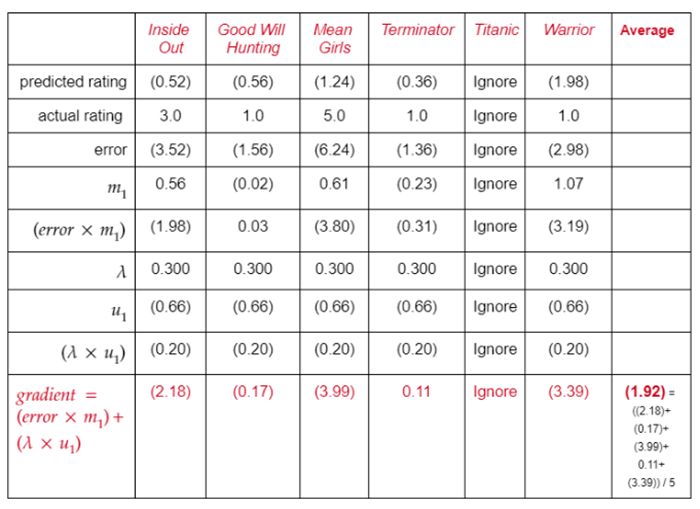### 更新权重

$$New \ u_1= old \ u_1-\alpha (average \ gradient)$$

$$New \ u_1=(0.66)-0.3(1.92)$$

$$New \ u_1=(0.66)+0.58$$

$$New \ u_1=(0.08)$$

“training”（训练）表的X11-X16单元格对应上面的计算过程。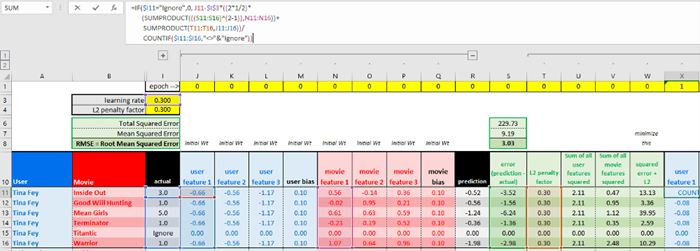## 第四部分：模型评估和可视化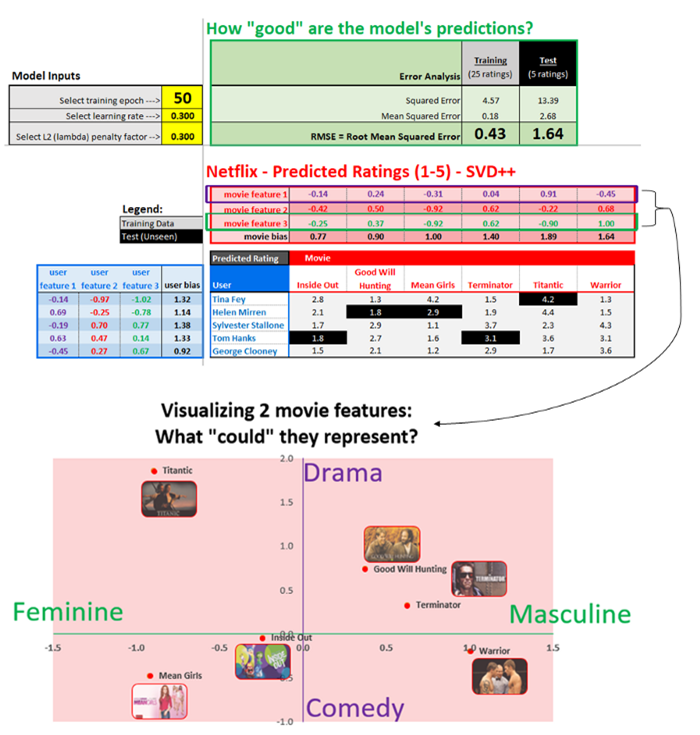## 总结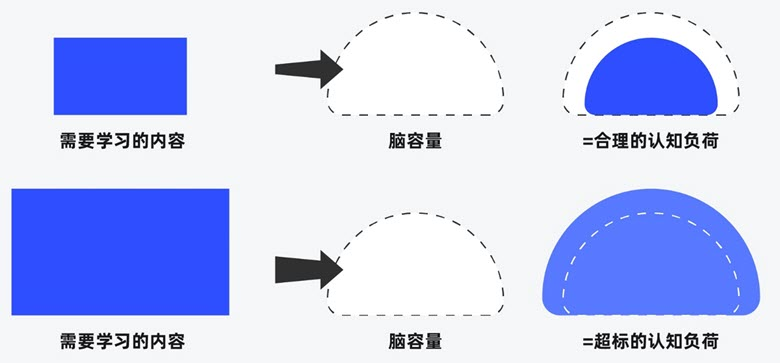##### 界面设计和游戏设计中的认知负荷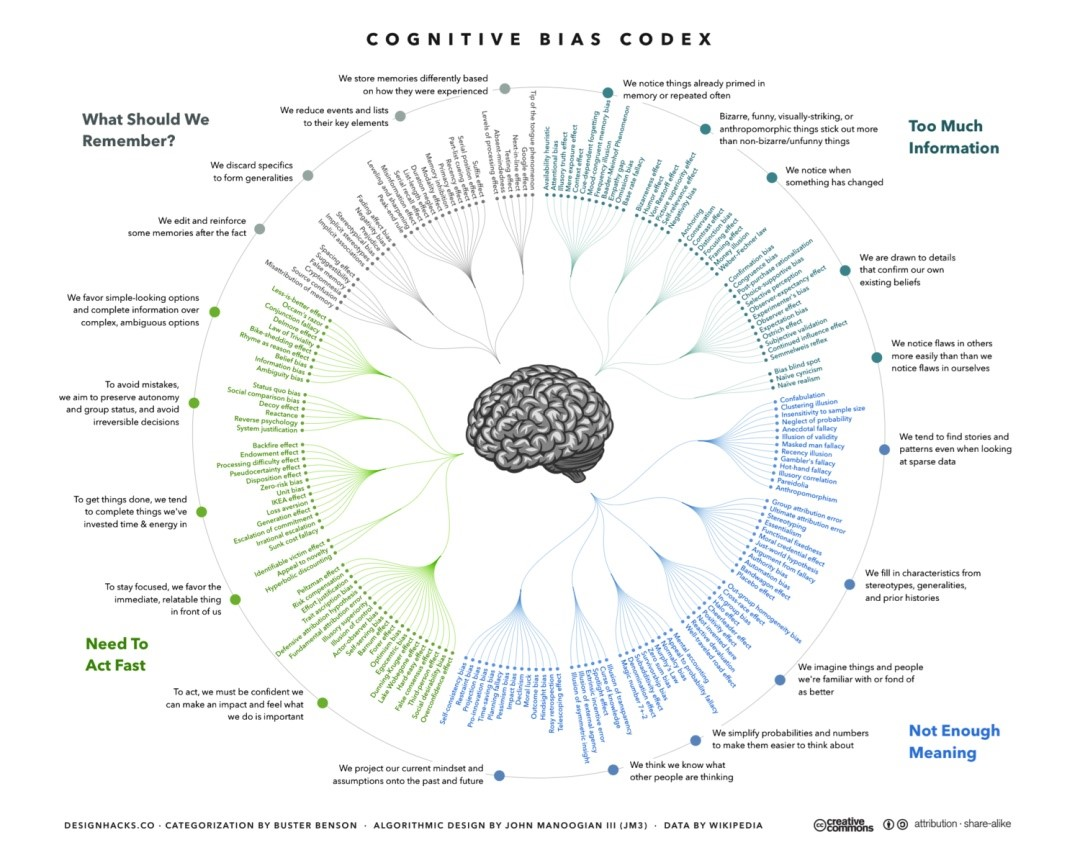##### 理解认知偏差